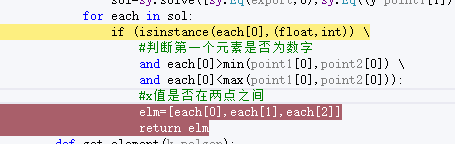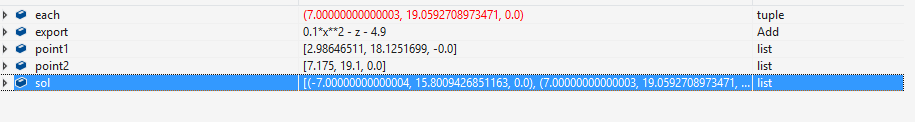# 新人求助，python中if语句判断未生效

``````            for each in sol:
if (isinstance(each,(float,int)) \
#判断第一个元素是否为数字
and each>min(point1,point2) \
and each<max(point1,point2)):
#x值是否在两点之间
elm=[each,each,each]
return elm
``````2019/04/18 01:32
• python
• 点赞
• 收藏
• 回答
私信

#### 1个回复